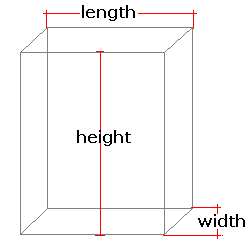Spike's Calculators

# Box Volume and Surface Area

Calculate the volume and surface area of a box, and include the girth measurement of the box.This calculator works for any measurement unit. For example, if you calculate inches, inches will have to be used in all the length fields, and the result of the areas will be square inches; the volume results in cubic inches and length measurements in the starting unit, inches.

Note:
`for the girth measurement the length is always considered the longest measurement. If your box shape has a longer height than the length measurement, the calculator will substitute height for length. And the width is always the shortest!`

### Box

Length of Box #
Width of Box #
Height of Box #
Decimal Precision #

#### Results:

 Box Volume #³ Bottom Area #² Top Area #² Lateral Area #² Surface Area #² Girth # Combined Length and Girth # Surface to Volume Ratio sa:vol

#### Calculation

• the length of the box
• the width of the box
• the height of the box
• decimal precision, number of digits after the decimal point

#### Results

• the volume of the box (unit cubed)
• the area of the bottom (unit squared)
• the area of the top (unit squared)
• the area of the four sides (lateral) (unit squared)
• the surface area = top + bottom + sides (unit squared)
• the girth of the box (starting unit)
• length plus girth (starting unit)
• surface area to volume ratio (decimal)
##### Formula
```V = LWH
bA = LW
tA = LW
lA = (L*H+W*H)2
sA = (L*W+L*H+W*H)2
s/v = sA/V
G = (W+H)2
where V is the volume of the box
bA the bottom area
tA the top area
lA the lateral surface area
sA the surface area
G the girth measurement of the box
s/v is the surface area to volume ratio
L = length
W = width
H = height
```